Скачать презентацию Dec 1 P Dec 2 W Evaluate 1 -3

4626d37d05332a6bcf16d36491707e77.ppt

• Количество слайдов: 22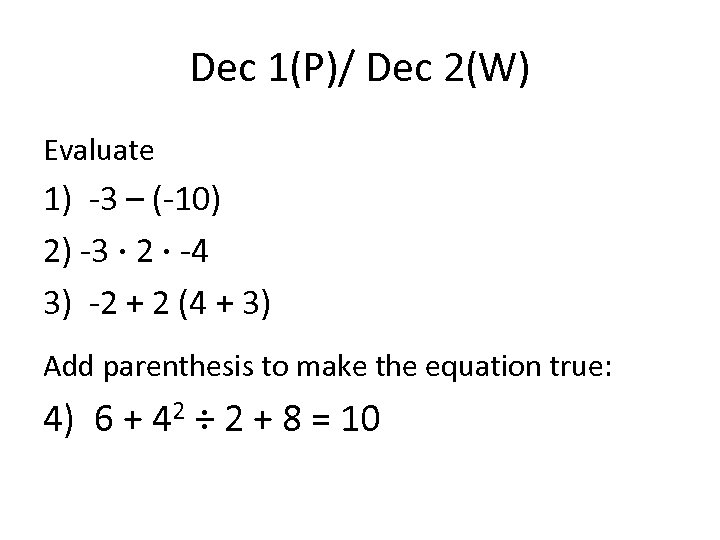Dec 1(P)/ Dec 2(W) Evaluate 1) -3 – (-10) 2) -3 · 2 · -4 3) -2 + 2 (4 + 3) Add parenthesis to make the equation true: 4) 6 + 42 ÷ 2 + 8 = 10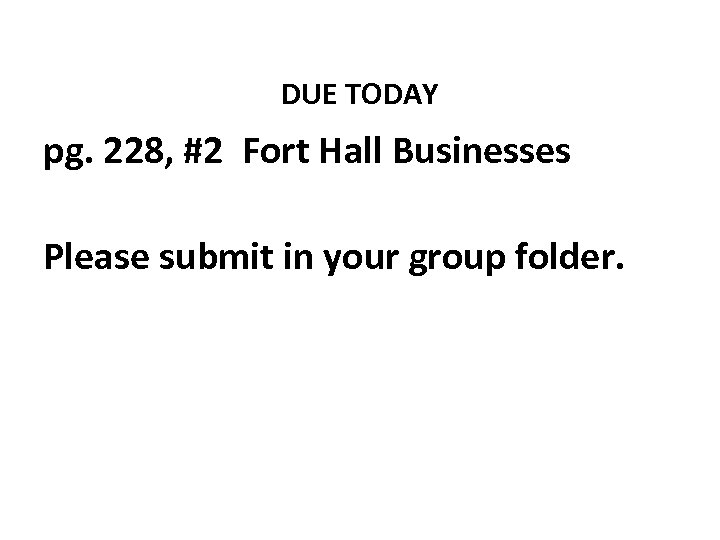DUE TODAY pg. 228, #2 Fort Hall Businesses Please submit in your group folder.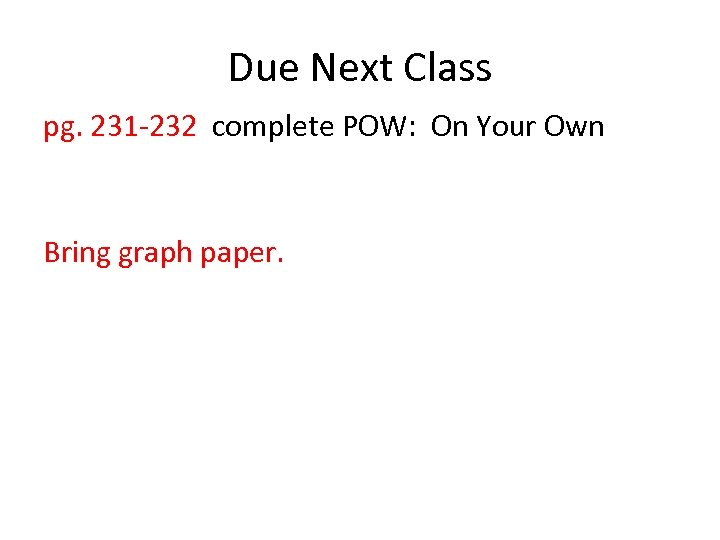Due Next Class pg. 231 -232 complete POW: On Your Own Bring graph paper.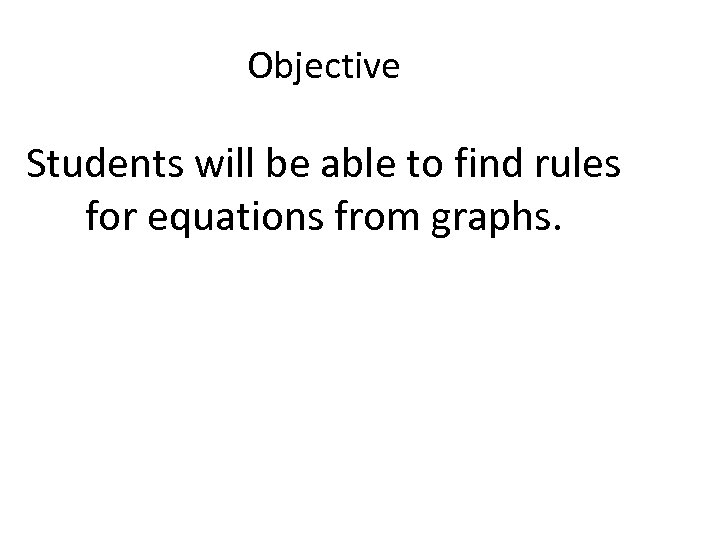Objective Students will be able to find rules for equations from graphs.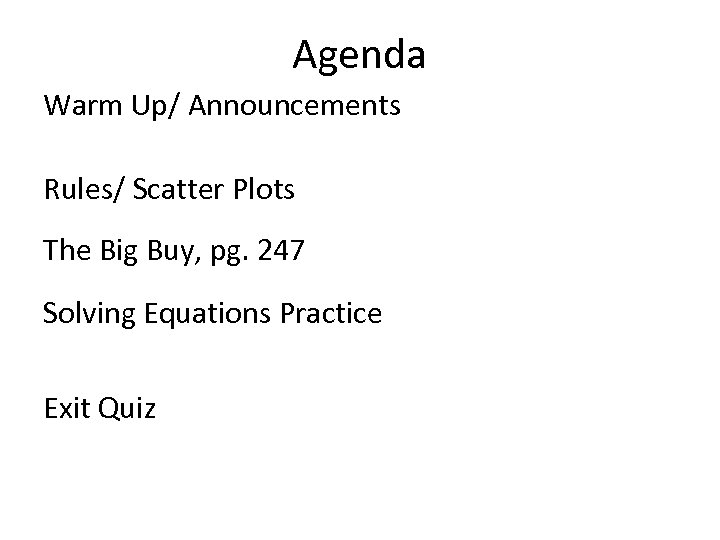Agenda Warm Up/ Announcements Rules/ Scatter Plots The Big Buy, pg. 247 Solving Equations Practice Exit Quiz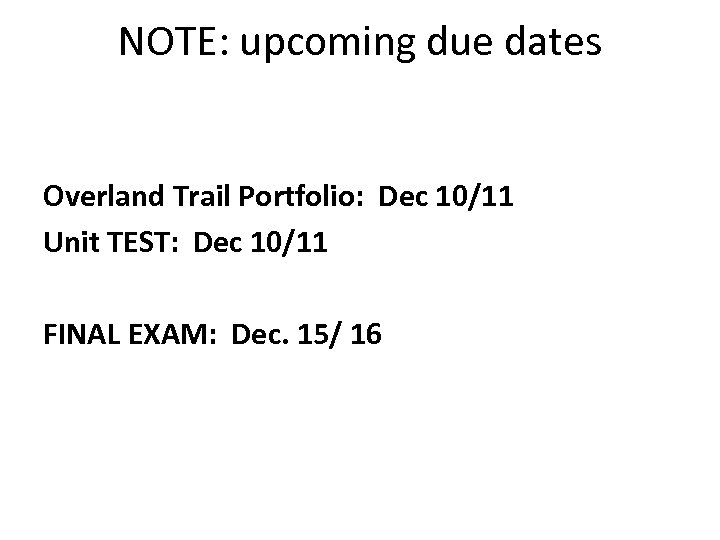NOTE: upcoming due dates Overland Trail Portfolio: Dec 10/11 Unit TEST: Dec 10/11 FINAL EXAM: Dec. 15/ 16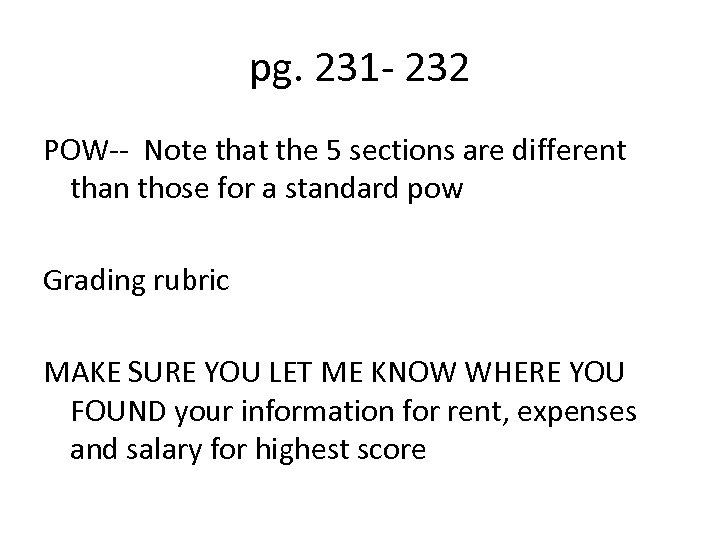pg. 231 - 232 POW-- Note that the 5 sections are different than those for a standard pow Grading rubric MAKE SURE YOU LET ME KNOW WHERE YOU FOUND your information for rent, expenses and salary for highest score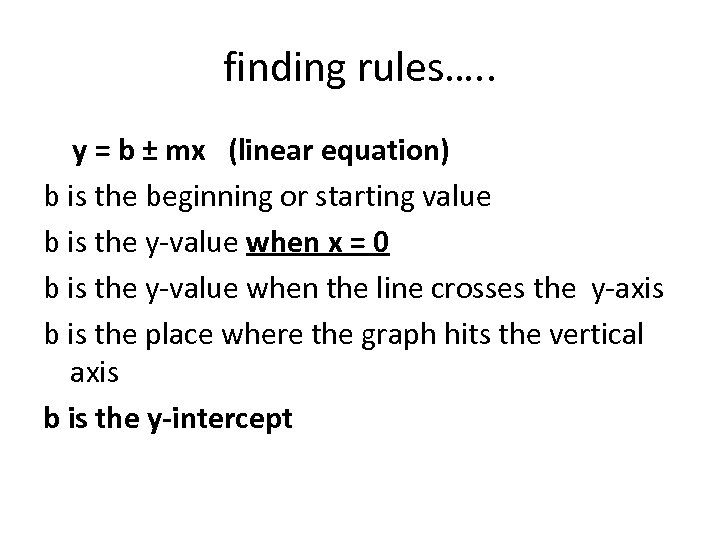finding rules…. . y = b ± mx (linear equation) b is the beginning or starting value b is the y-value when x = 0 b is the y-value when the line crosses the y-axis b is the place where the graph hits the vertical axis b is the y-intercept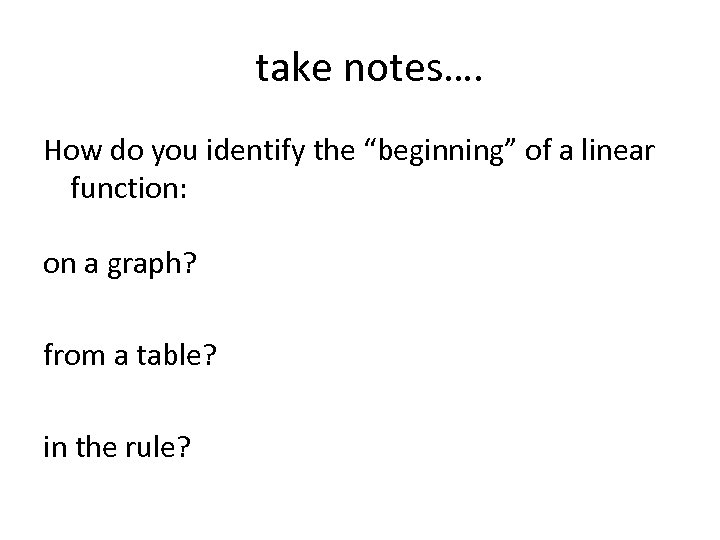take notes…. How do you identify the “beginning” of a linear function: on a graph? from a table? in the rule?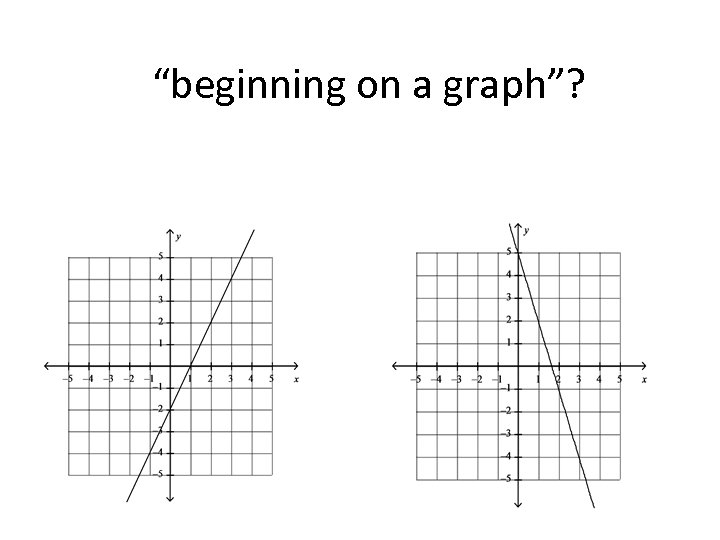“beginning on a graph”?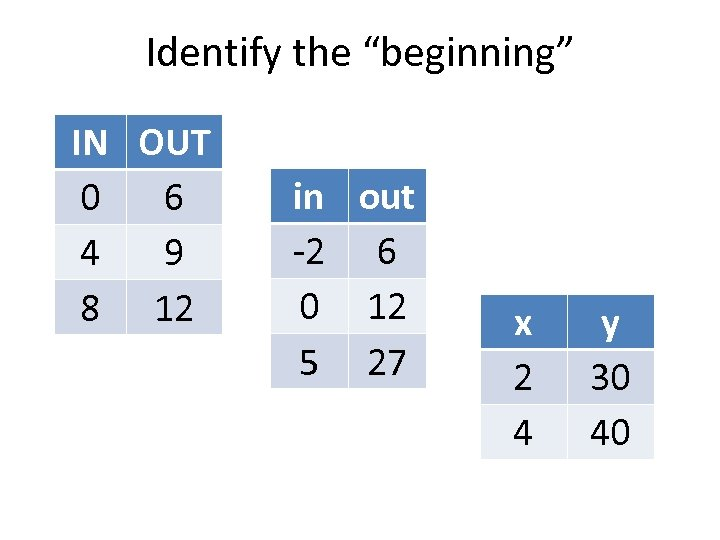Identify the “beginning” IN OUT 0 6 4 9 8 12 in out -2 6 0 12 5 27 x 2 4 y 30 40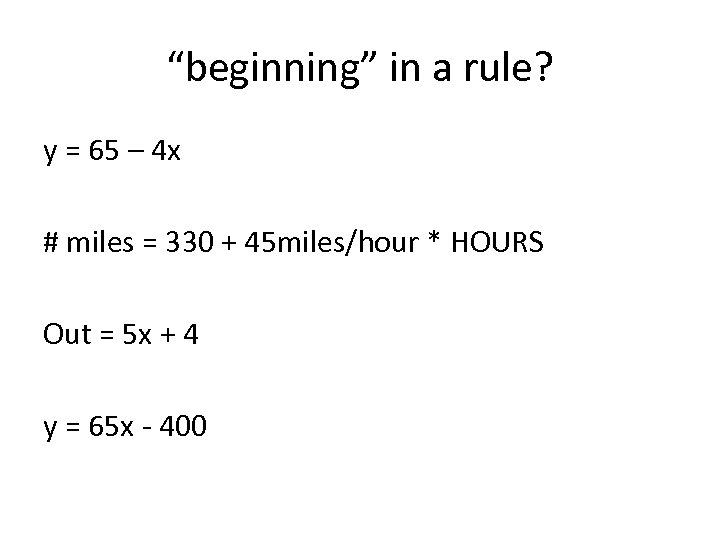“beginning” in a rule? y = 65 – 4 x # miles = 330 + 45 miles/hour * HOURS Out = 5 x + 4 y = 65 x - 400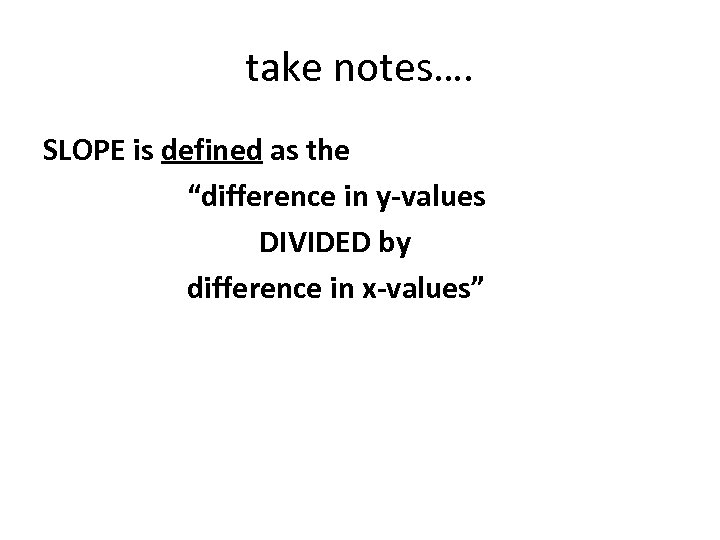take notes…. SLOPE is defined as the “difference in y-values DIVIDED by difference in x-values”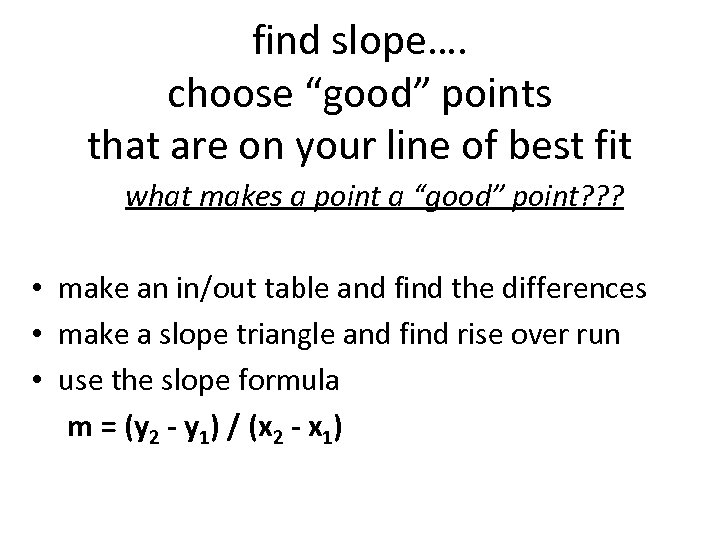find slope…. choose “good” points that are on your line of best fit what makes a point a “good” point? ? ? • make an in/out table and find the differences • make a slope triangle and find rise over run • use the slope formula m = (y 2 - y 1) / (x 2 - x 1)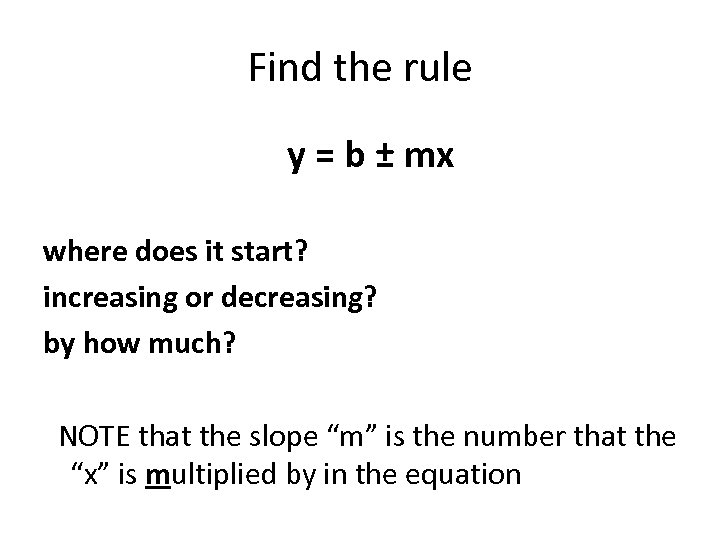Find the rule y = b ± mx where does it start? increasing or decreasing? by how much? NOTE that the slope “m” is the number that the “x” is multiplied by in the equation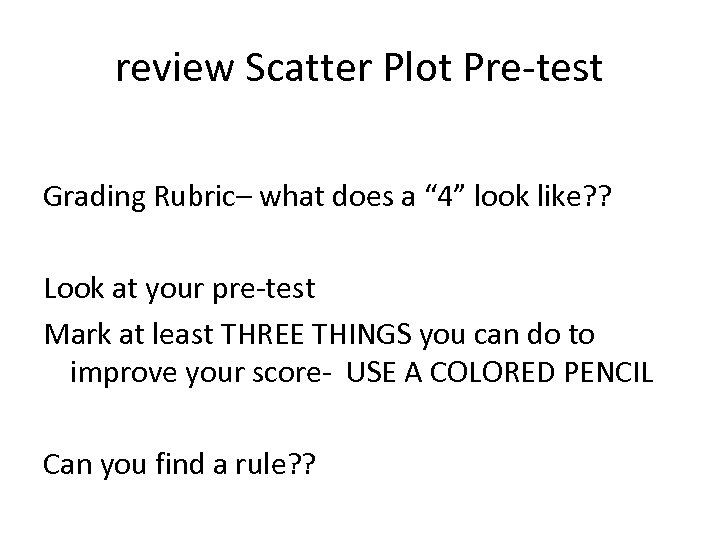review Scatter Plot Pre-test Grading Rubric– what does a “ 4” look like? ? Look at your pre-test Mark at least THREE THINGS you can do to improve your score- USE A COLORED PENCIL Can you find a rule? ?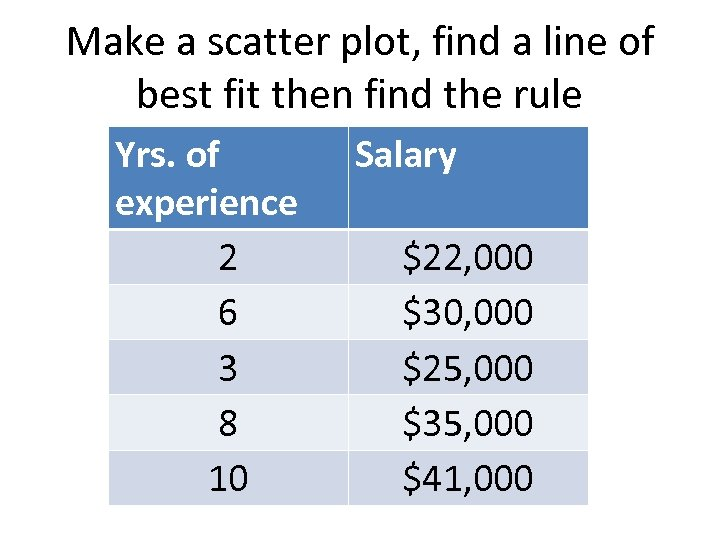Make a scatter plot, find a line of best fit then find the rule Yrs. of experience 2 6 3 8 10 Salary \$22, 000 \$30, 000 \$25, 000 \$35, 000 \$41, 000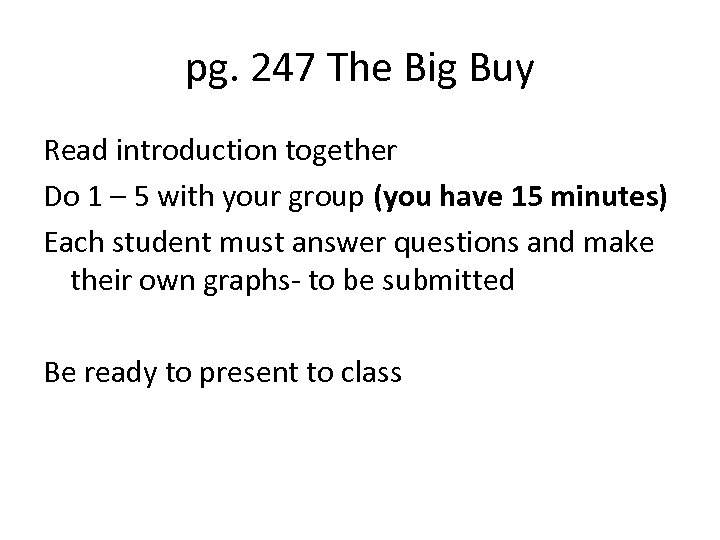pg. 247 The Big Buy Read introduction together Do 1 – 5 with your group (you have 15 minutes) Each student must answer questions and make their own graphs- to be submitted Be ready to present to class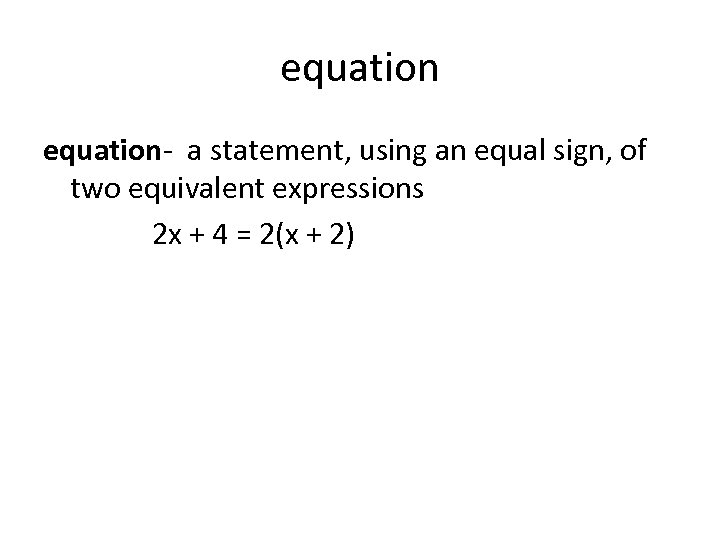equation- a statement, using an equal sign, of two equivalent expressions 2 x + 4 = 2(x + 2)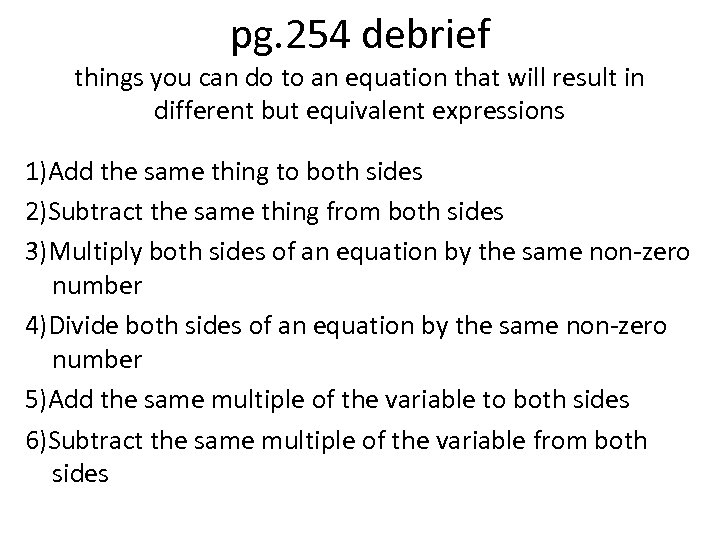pg. 254 debrief things you can do to an equation that will result in different but equivalent expressions 1)Add the same thing to both sides 2)Subtract the same thing from both sides 3)Multiply both sides of an equation by the same non-zero number 4)Divide both sides of an equation by the same non-zero number 5)Add the same multiple of the variable to both sides 6)Subtract the same multiple of the variable from both sides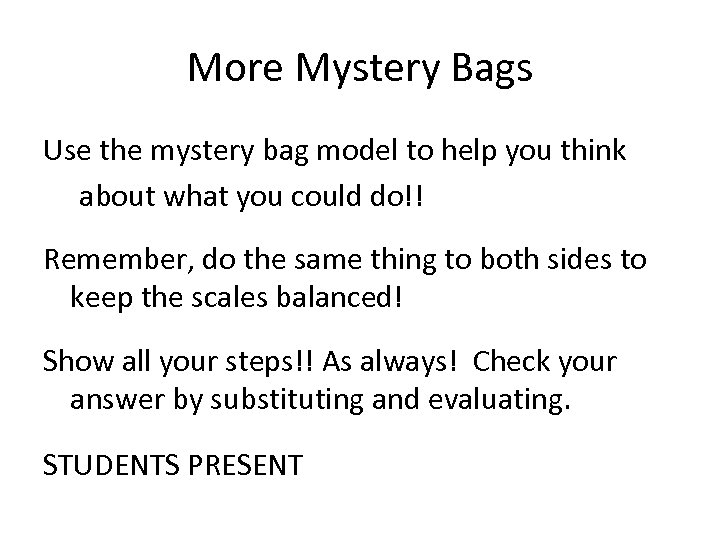More Mystery Bags Use the mystery bag model to help you think about what you could do!! Remember, do the same thing to both sides to keep the scales balanced! Show all your steps!! As always! Check your answer by substituting and evaluating. STUDENTS PRESENTEXIT QUIZ Put books away. Put WU and CW in left side of group folders. You may keep a calculator and ruler. Complete scatter plot quiz. I will collect them at the end of class.